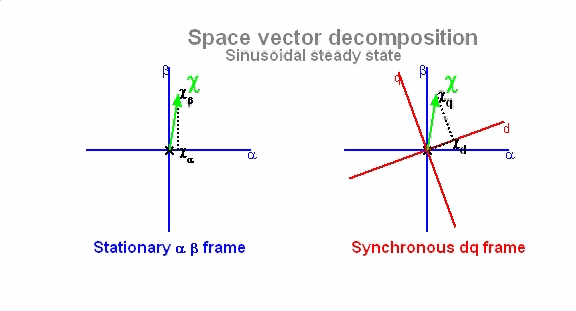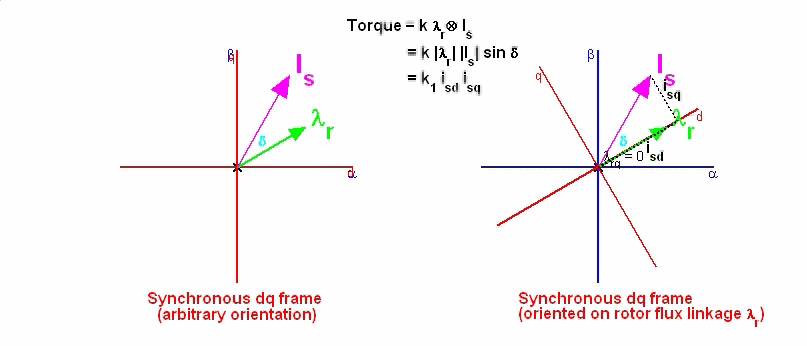﻿ Space vector decomposition

SPACE VECTOR DECOMPOSITION

This demo animates the motion of space vectors under balanced sinusoidal conditions, appearing as constant amplitude vectors rotating at the excitation frequency. The components depend on the choice of reference frame. In the stationary αβ frame, the components are time varying representing two-phase sinusoidal signals at stator frequency. In the rotating synchronous dq frame, the dq components are constant whose values depend on the orientation of the space vectors with respect to the dq axes. The state vectors Is (stator current) and λr (rotor flux linkage) are shown in the common dq synchronous frame. When the d_axis is aligned with the rotor field --a process referred to as field orientation--, λrq = 0 and the torque is expressed as Te = k1 isd isq. The current isd   is the field component and isq is the torque component of the stator current space vector Is.